# Divisibility Rules

Divisibility Rules

In this mini-lesson, we will explore about divisibility rules by learning how to apply divisibility rules with examples, and the divisibility rules of specific numbers while discovering the interesting facts around them.

In a 1962 Scientific American article, the popular science writer, Martin Gardner, discusses divisibility rules for 2–12, where he explains that the rules were widely known during the renaissance and used to reduce fractions with large numbers down to the lowest terms.

Since every number is not completely divisible by every other number, they may leave a remainder other than zero. There are certain rules which help us determine the actual divisor of a number just by considering the digits of that number. These are called divisibility rules.

Do you think, 375 is divisible 6?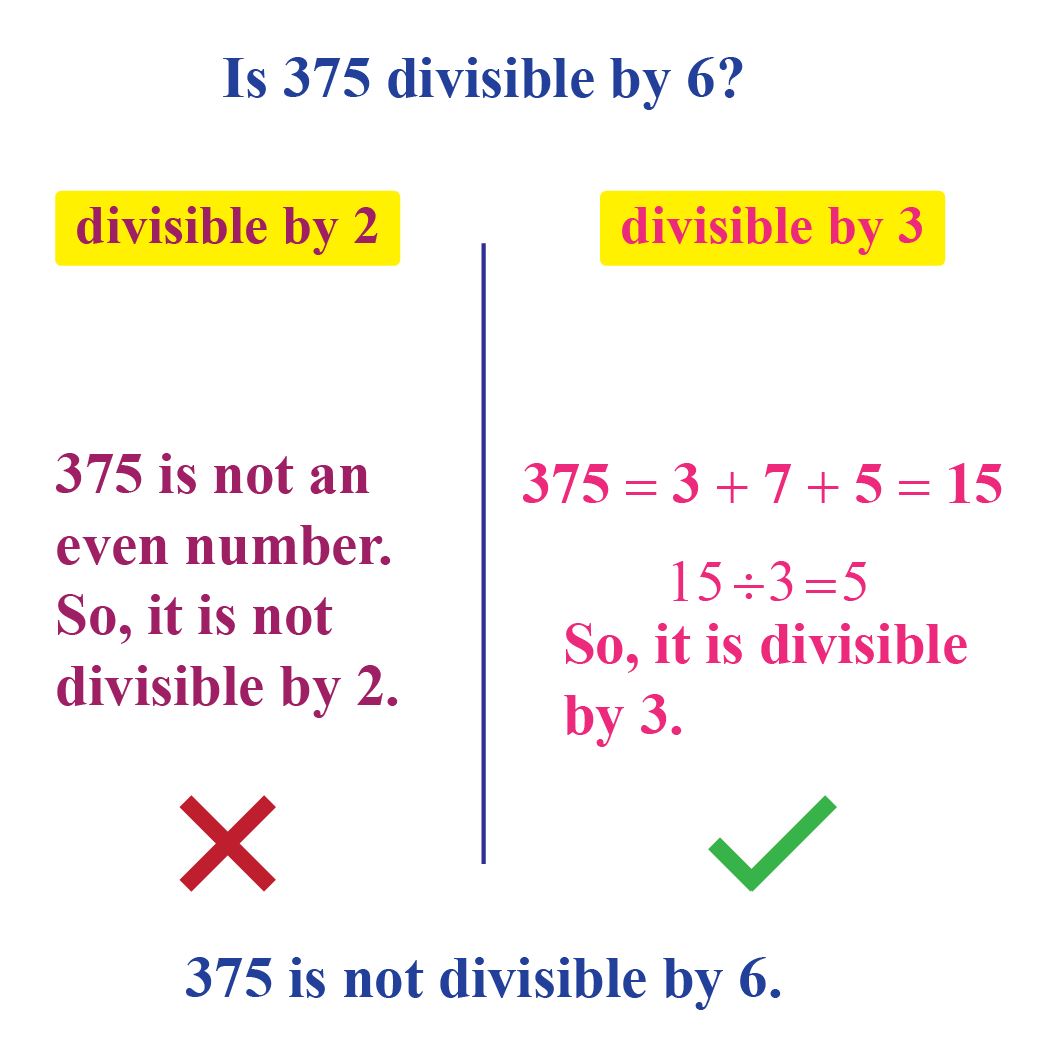Let us all find answers to all such questions in this lesson on divisibility rules!

## Lesson Plan

 1 What Are Divisibility Rules? 2 Solved Examples on Divisibility Rules 3 Interactive Questions on Divisibility Rules

## What Are Divisibility Rules?

A divisibility rule is a kind of shortcut to figure out if a given integer is divisible by a divisor, without performing the whole division process but by examining its digits.

Multiple divisibility rules can be applied to the same number which can quickly determine its prime factorization.

Divisibility rules are a set of general rules or heuristics that are used to figure out if a number is wholly divisible by another number.

## What Are the Basic Divisibility Rules?

Here are a few basic divisibility rules:

Divisibility by number Divisibility Rule
Divisible by 2 A number is even or a number whose last digit is an even number i.e. 2, 4, 6, 8 including 0
Divisible by 3 The sum of the digits of the number is divisible by 3
Divisible by 4 The last two digits of the number are divisible by 4
Divisible by 5 A number which has 0 or 5 as their last digits
Divisible by 6 A number which is divisible by both 2 and 3
Divisible by 7 Subtracting twice the last digit of the number from the remaining digits gives a multiple of 7
Divisible by 8 The last three digits of a number are divisible by 8
Divisible by 9 The sum of the digits of the number is divisible by 9
Divisible by 10 Any number whose last digit is 0
Divisible by 11 The difference of the sum of the alternative digits of a number is divisible by 11
Divisible by 12 A number which is divisible by both 3 and 4

Examples

1)2)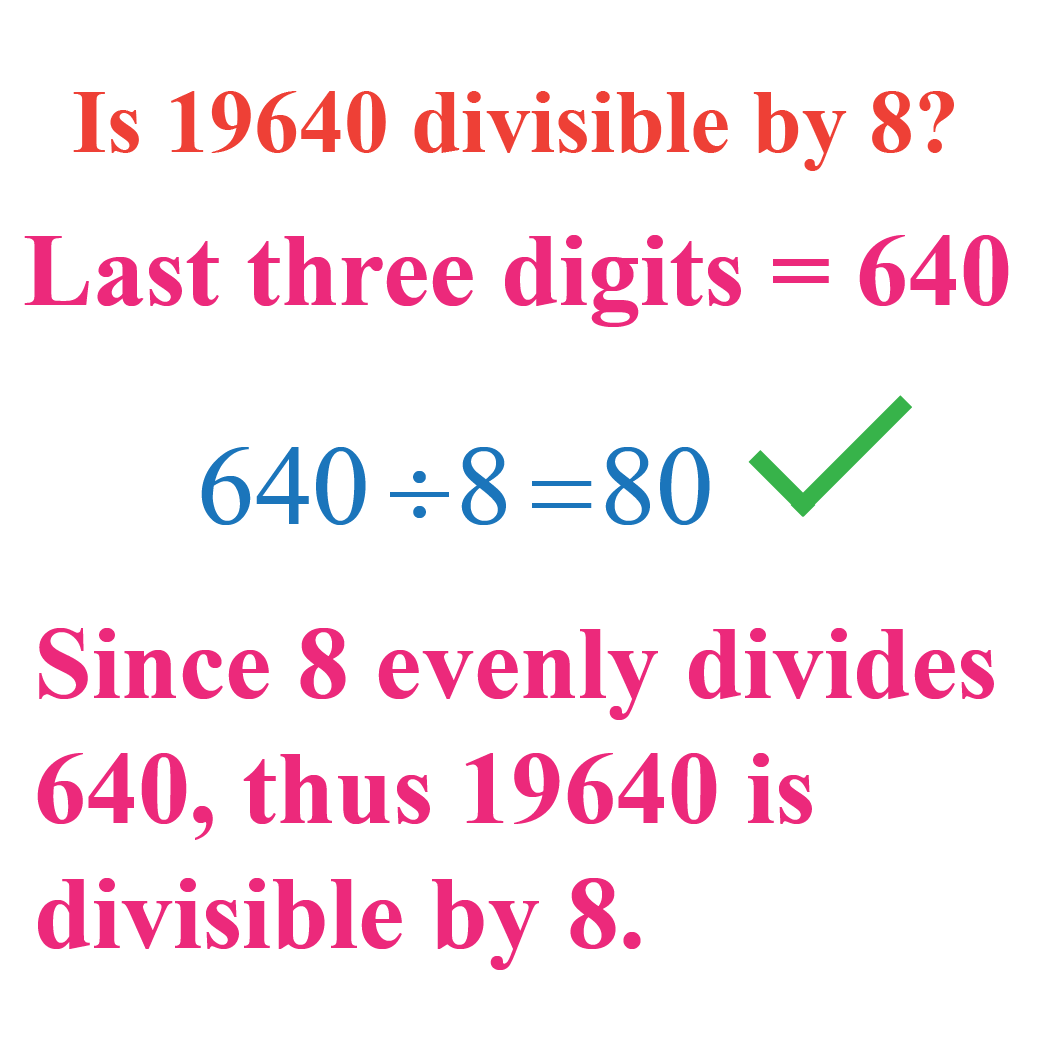3)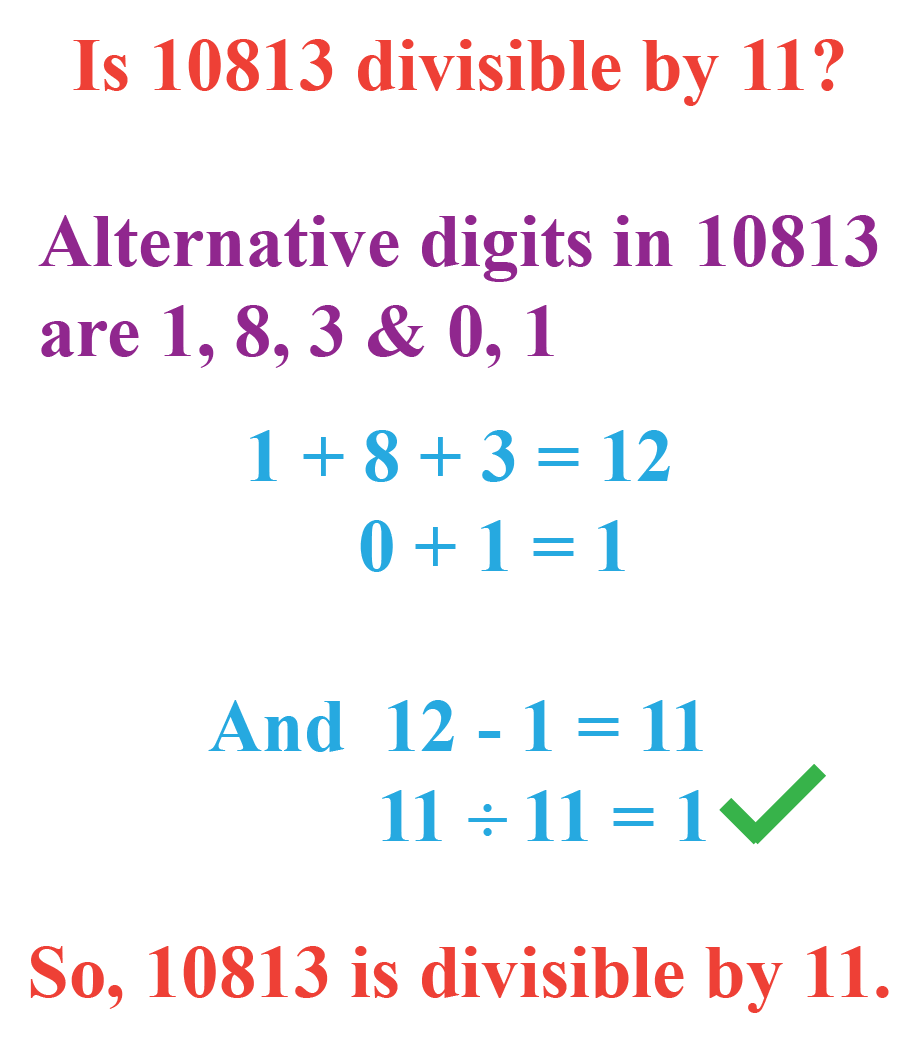## What Are Intermediate Divisibility Rules?

Intermediate divisibility rules are applied to prime numbers which are less than 20 and greater than 10.

Divisibility by number Divisibility Rule
Divisibility by  13  If 4 times the units digit of the number plus the number obtained by removing the units digits of the number is a multiple of 13
Divisibility by 17  If the units digit of a number is subtracted 5 times from the remaining (excluding the units digit) results in a number that is divisibly by 17
Divisibility by 19  If doubling the units digit and adding it to the number formed by removing the units digits in the original number is divisible by 19

Examples

1)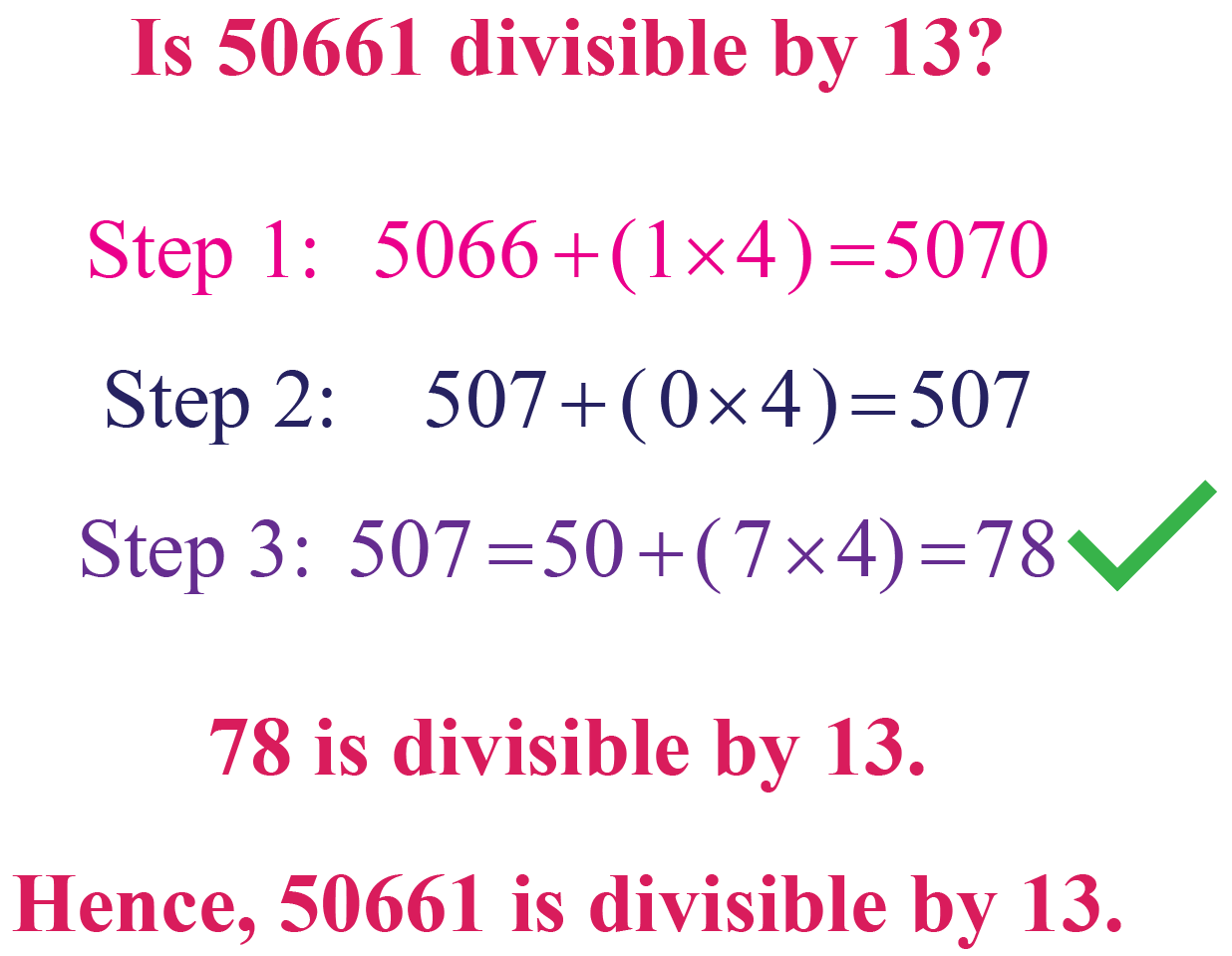2)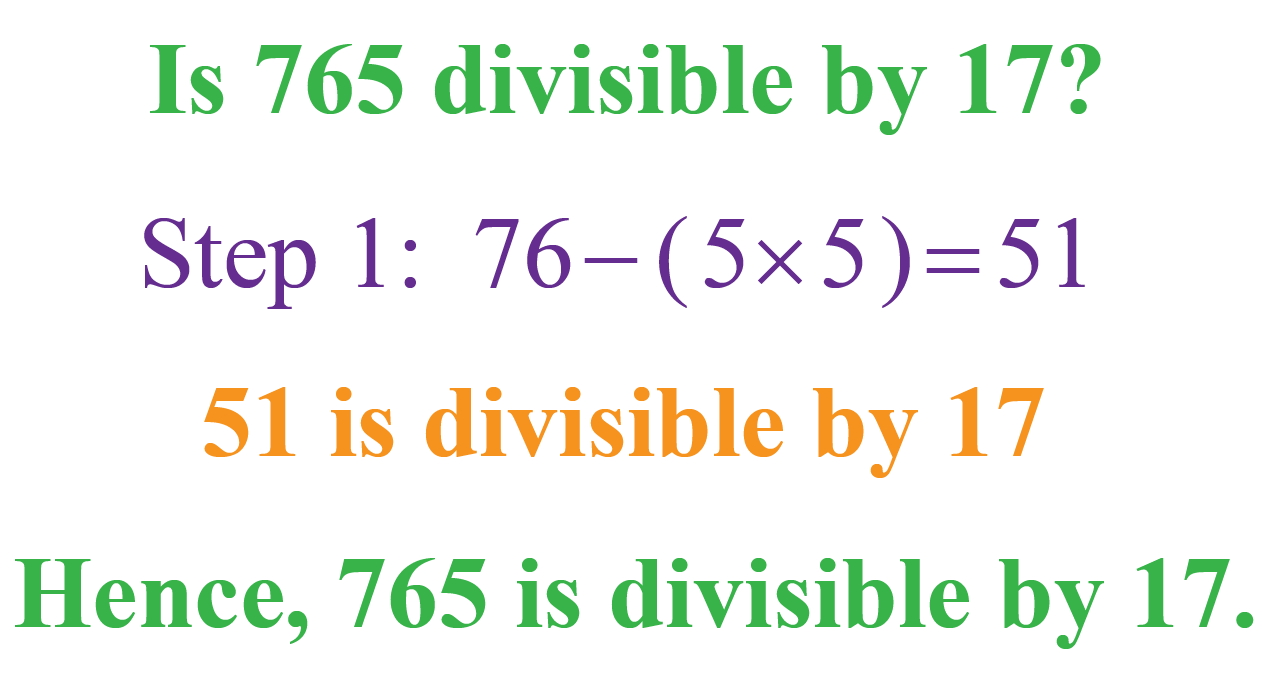3)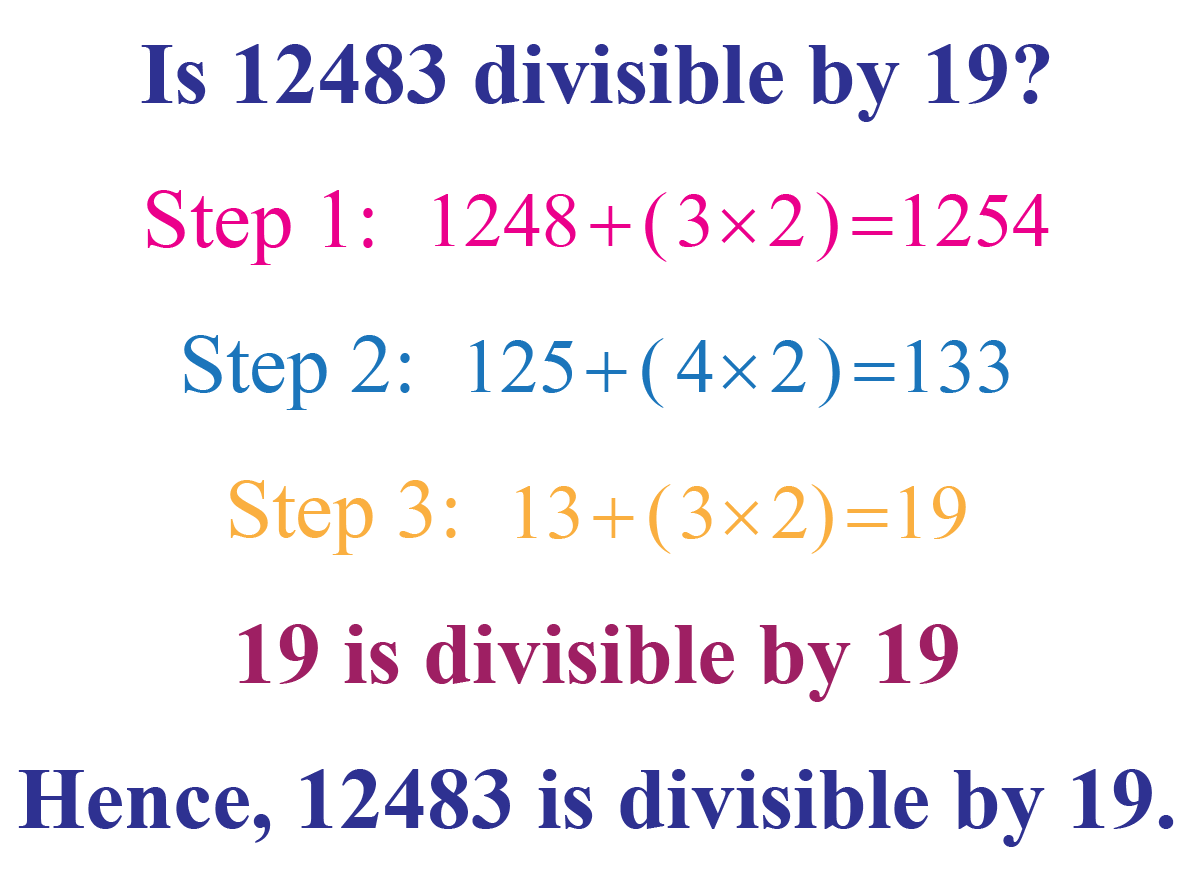Think Tank
1. A number is divisible by 4 and 12. Is it true that it will be divisible by 48?
2. Check whether 2359334 follow divisibility rules of 4 as well as 8

## How to Prove Divisibility Rules for 7 and 8?

### Divisibility Rule for 7

Explanation

Step 1: Multiply the units digit by 2

Step 2: Subtract the product from the remaining digits.

Step 3: Check the difference.

If the difference is divisible by 7, then the number is divisible by 7

For example: Is 623 divisible by 7?

Step 1: 3 x 2 = 6
Step 2: 62 – 6 = 56
Step 3: The difference is 56 and 56 is divisible by 7
Thus, 623 is divisible by 7

### Divisibility Rule for 8

Explanation

A number is divisible by 8 when the last three digits are evenly divisible by 8

For example, check if 17224 is divisible by 8

The last three digits are 224 and the number 224 is divisible by 8. Hence, 17224 is divisible by 8

More Important Topics
Numbers
Algebra
Geometry
Measurement
Money
Data
Trigonometry
Calculus